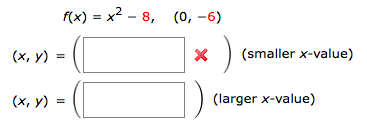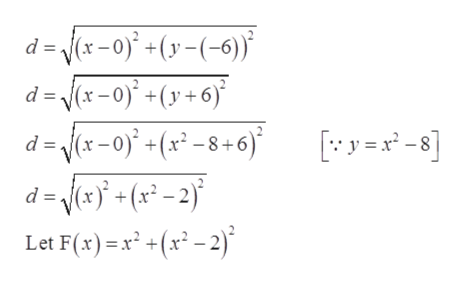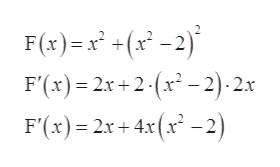# f(x) - х2 - 8, (0, —6)(smaller x-value)(х, у)(x, y) =(larger x-value)%3D

Question
4 views

Find the points on the graph of the function that are closest to the given point.help_outlineImage Transcriptionclosef(x) - х2 - 8, (0, —6) (smaller x-value) (х, у) (x, y) = (larger x-value) %3D fullscreen
check_circle

Step 1

Given:

Step 2

Distance from point (0, -6) and (x, y) is:help_outlineImage Transcriptionclosed = (x-o0)° +(y-(-6)) d= (x-0)° +(y+6) d = (x-0)* +(x` -8+6) d = (x} +(x° - 2)° [y =x* -8] Let F(x) = x² +(x² – 2)* fullscreen
Step 3

Differentiate F(x) with respe...help_outlineImage TranscriptioncloseF(x)=x° +(x° -2)* F'(x) = 2x + 2-(x² – 2)· 2.x F'(x) = 2x + 4.x(x² - 2) fullscreen

### Want to see the full answer?

See Solution

#### Want to see this answer and more?

Solutions are written by subject experts who are available 24/7. Questions are typically answered within 1 hour.*

See Solution
*Response times may vary by subject and question.
Tagged in

### Derivative